SLVS919C January   2009  – September 2015

PRODUCTION DATA.

1. Features
2. Applications
3. Description
4. Revision History
5. Pin Configuration and Functions
6. Specifications
7. Detailed Description
8. Application and Implementation
1. 8.1 Application Information
2. 8.2 Typical Applications
1. 8.2.1 Buck Converter for 3.3-V Output
2. 8.2.2 Inverting Power Supply
3. 8.2.3 Split Rail Power Supply
9. Power Supply Recommendations
10. 10Layout
1. 10.1 Layout Guidelines
2. 10.2 Layout Example
11. 11Device and Documentation Support
1. 11.1 Device Support
2. 11.2 Documentation Support
4. 11.4 Electrostatic Discharge Caution
5. 11.5 Glossary
12. 12Mechanical, Packaging, and Orderable Information

• DGQ|10
• DRC|10
• DGQ|10
• DRC|10

8 Application and Implementation

NOTE

Information in the following applications sections is not part of the TI component specification, and TI does not warrant its accuracy or completeness. TI’s customers are responsible for determining suitability of components for their purposes. Customers should validate and test their design implementation to confirm system functionality.

8.1 Application Information

The TPS54060 device is a 60-V, 0.5-A, step-down regulator with an integrated high-side MOSFET. This device typically converts a higher dc voltage to a lower dc voltage with a maximum available output current of 0.5 A. Example applications are: 12-V, 24-V, and 48-V industrial, automotive and communication power systems. Use the following design procedure to select component values for the TPS54060 device. The Excel® spreadsheet (SLVC432) located on the product page can help on all calculations. Alternatively, use the WEBENCH software to generate a complete design. The WEBENCH software uses an iterative design procedure and accesses a comprehensive database of components when generating a design.

The output voltage is set with a resistor divider from the output node to the VSENSE pin. It is recommended to use 1% tolerance or better divider resistors. Start with a 10 kΩ for the R2 resistor and use the Equation 17 to calculate R1. To improve efficiency at light loads consider using larger value resistors. If the values are too high the regulator will be more susceptible to noise and voltage errors from the VSENSE input current will be noticeable

Equation 17.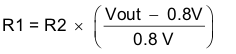8.1.2 Enable and Adjusting Undervoltage Lockout

The TPS54060 is disabled when the VIN pin voltage falls below 2.5 V. If an application requires a higher undervoltage lockout (UVLO), use the EN pin as shown in Figure 35 to adjust the input voltage UVLO by using the two external resistors. Though it is not necessary to use the UVLO adjust registers, for operation it is highly recommended to provide consistent power up behavior. The EN pin has an internal pull-up current source, I1, of 0.9μA that provides the default condition of the TPS54060 operating when the EN pin floats. Once the EN pin voltage exceeds 1.25V, an additional 2.9μA of hysteresis, Ihys, is added. This additional current facilitates input voltage hysteresis. Use Equation 18 to set the external hysteresis for the input voltage. Use Equation 19 to set the input start voltage.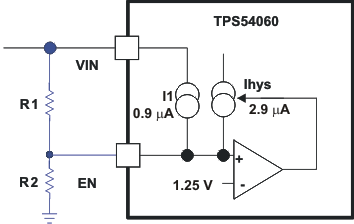Figure 35. Adjustable Undervoltage Lockout (UVLO)
Equation 18.Equation 19.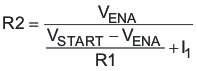Another technique to add input voltage hysteresis is shown in Figure 36. This method may be used, if the resistance values are high from the previous method and a wider voltage hysteresis is needed. The resistor R3 sources additional hysteresis current into the EN pin.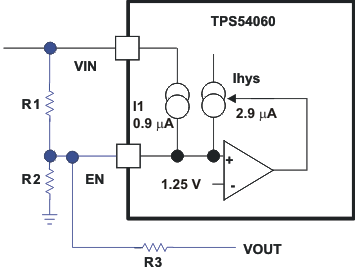Figure 36. Adding Additional Hysteresis
Equation 20.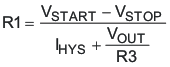Equation 21.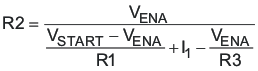8.1.3 Sequencing

Many of the common power supply sequencing methods can be implemented using the SS/TR, EN and PWRGD pins. The sequential method can be implemented using an open drain output of a power on reset pin of another device. The sequential method is illustrated in Figure 37 using two TPS54060 devices. The power good is coupled to the EN pin on the TPS54060 which will enable the second power supply once the primary supply reaches regulation. If needed, a 1nF ceramic capacitor on the EN pin of the second power supply will provide a 1ms start up delay. Figure 38 shows the results of Figure 37.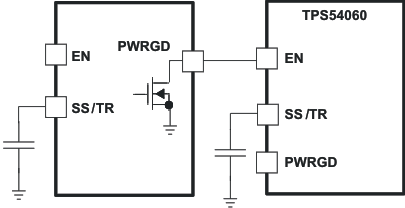Figure 37. Schematic for Sequential Start-Up Sequence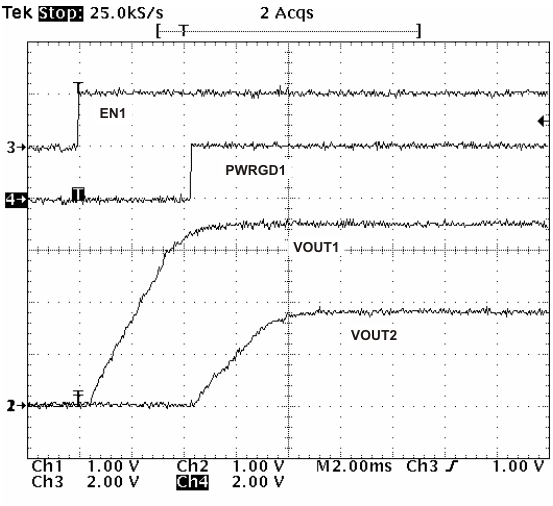Figure 38. Sequential Startup Using EN and PWRGD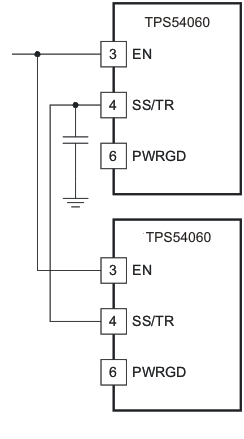Figure 39. Schematic for Ratiometric Start-Up Sequence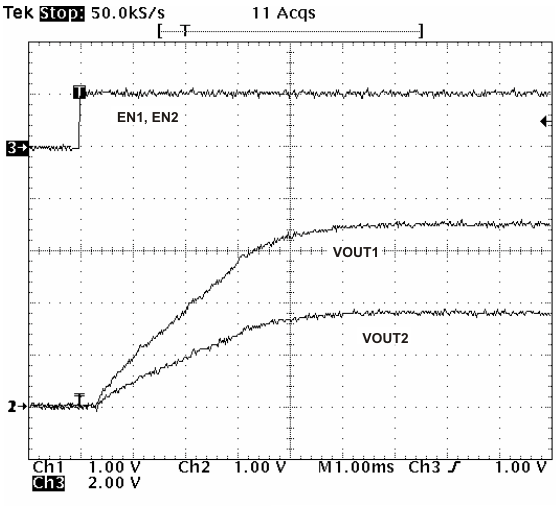Figure 40. Ratiometric Start-Up Using Coupled SS/TR Pins

Figure 39 shows a method for ratio-metric start up sequence by connecting the SS/TR pins together. The regulator outputs will ramp up and reach regulation at the same time. When calculating the slow start time the pull up current source must be doubled in Equation 1. Figure 40 shows the results of Figure 39.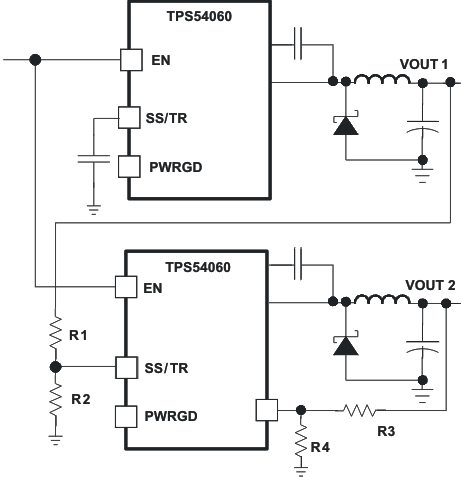Figure 41. Schematic for Ratiometric and Simultaneous Start-Up Sequence

Ratio-metric and simultaneous power supply sequencing can be implemented by connecting the resistor network of R1 and R2 shown in Figure 41 to the output of the power supply that needs to be tracked or another voltage reference source. Using Equation 22 and Equation 23, the tracking resistors can be calculated to initiate the Vout2 slightly before, after or at the same time as Vout1. Equation 24 is the voltage difference between Vout1 and Vout2 at the 95% of nominal output regulation.

The deltaV variable is zero volts for simultaneous sequencing. To minimize the effect of the inherent SS/TR to VSENSE offset (Vssoffset) in the slow start circuit and the offset created by the pullup current source (Iss) and tracking resistors, the Vssoffset and Iss are included as variables in the equations.

To design a ratio-metric start up in which the Vout2 voltage is slightly greater than the Vout1 voltage when Vout2 reaches regulation, use a negative number in Equation 22 through Equation 24 for deltaV. Equation 24 will result in a positive number for applications which the Vout2 is slightly lower than Vout1 when Vout2 regulation is achieved.

Since the SS/TR pin must be pulled below 40mV before starting after an EN, UVLO or thermal shutdown fault, careful selection of the tracking resistors is needed to ensure the device will restart after a fault. Make sure the calculated R1 value from Equation 22 is greater than the value calculated in Equation 25 to ensure the device can recover from a fault.

As the SS/TR voltage becomes more than 85% of the nominal reference voltage the Vssoffset becomes larger as the slow start circuits gradually handoff the regulation reference to the internal voltage reference. The SS/TR pin voltage needs to be greater than 1.3V for a complete handoff to the internal voltage reference as shown in Figure 23.

Equation 22.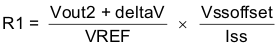Equation 23.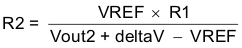Equation 24.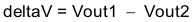Equation 25.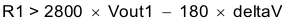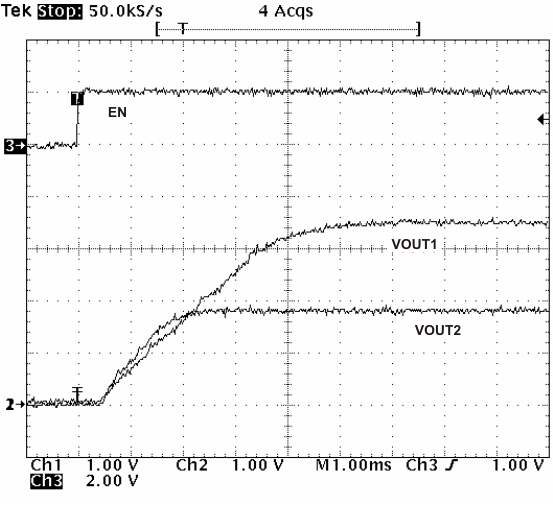Figure 42. Ratiometric Startup With Tracking Resistors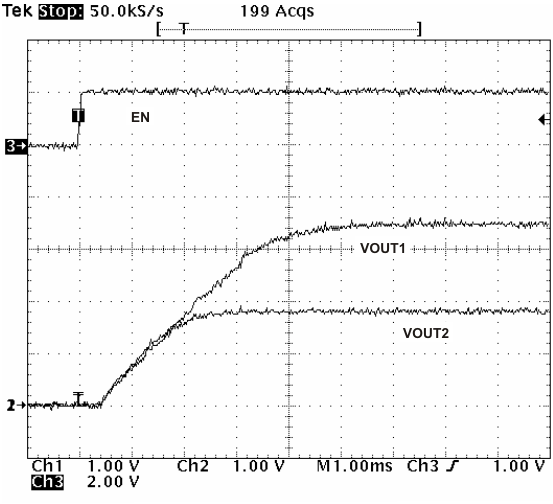Figure 44. Simultaneous Startup With Tracking Resistor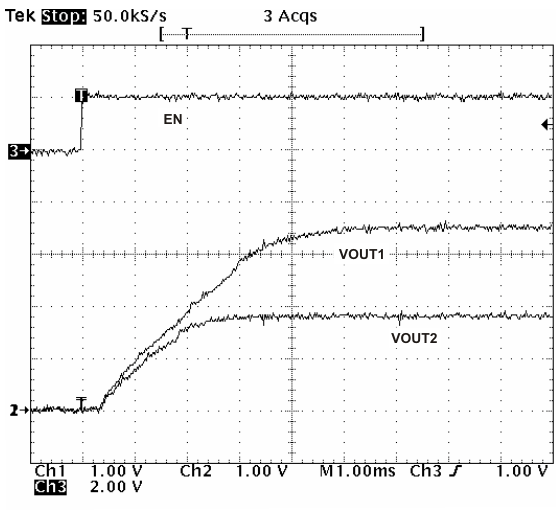Figure 43. Ratiometric Startup With Tracking Resistors

8.1.4 Selecting the Switching Frequency

The switching frequency that is selected should be the lower value of the two equations, Equation 26 and Equation 27. Equation 26 is the maximum switching frequency limitation set by the minimum controllable on time. Setting the switching frequency above this value will cause the regulator to skip switching pulses.

Equation 27 is the maximum switching frequency limit set by the frequency shift protection. To have adequate output short circuit protection at high input voltages, the switching frequency should be set to be less than the fsw(maxshift) frequency. In Equation 27, to calculate the maximum switching frequency one must take into account that the output voltage decreases from the nominal voltage to 0 volts, the fdiv integer increases from 1 to 8 corresponding to the frequency shift.

In Figure 45, the solid line illustrates a typical safe operating area regarding frequency shift and assumes the output voltage is zero volts, and the resistance of the inductor is 0.130Ω, FET on resistance of 0.2Ω and the diode voltage drop is 0.5V. The dashed line is the maximum switching frequency to avoid pulse skipping. Enter these equations in a spreadsheet or other software or use the SwitcherPro design software to determine the switching frequency.

Equation 26.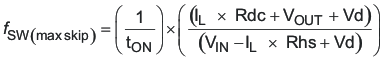Equation 27.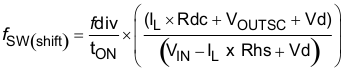IL inductor current Rdc inductor resistance VIN maximum input voltage VOUT output voltage VOUTSC output voltage during short Vd diode voltage drop RDS(on) switch on resistance tON controllable on time ƒDIV frequency divide equals (1, 2, 4, or 8)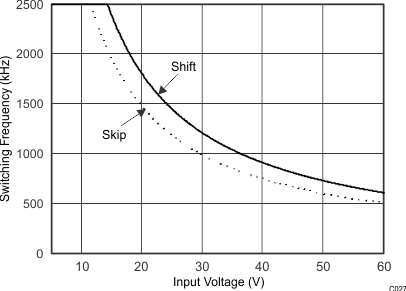Figure 45. Maximum Switching Frequency vs Input Voltage

8.1.5 How to Interface to RT/CLK Pin

The RT/CLK pin can be used to synchronize the regulator to an external system clock. To implement the synchronization feature connect a square wave to the RT/CLK pin through the circuit network shown in Figure 46. The square wave amplitude must transition lower than 0.5V and higher than 2.2V on the RT/CLK pin and have an on time greater than 40 ns and an off time greater than 40 ns. The synchronization frequency range is 300 kHz to 2200 kHz. The rising edge of the PH will be synchronized to the falling edge of RT/CLK pin signal. The external synchronization circuit should be designed in such a way that the device will have the default frequency set resistor connected from the RT/CLK pin to ground should the synchronization signal turn off. It is recommended to use a frequency set resistor connected as shown in Figure 46 through a 50Ω resistor to ground. The resistor should set the switching frequency close to the external CLK frequency. It is recommended to ac couple the synchronization signal through a 10 pF ceramic capacitor to RT/CLK pin and a 4kΩ series resistor. The series resistor reduces PH jitter in heavy load applications when synchronizing to an external clock and in applications which transition from synchronizing to RT mode. The first time the CLK is pulled above the CLK threshold the device switches from the RT resistor frequency to PLL mode. The internal 0.5V voltage source is removed and the CLK pin becomes high impedance as the PLL starts to lock onto the external signal. Since there is a PLL on the regulator the switching frequency can be higher or lower than the frequency set with the external resistor. The device transitions from the resistor mode to the PLL mode and then will increase or decrease the switching frequency until the PLL locks onto the CLK frequency within 100 microseconds.

When the device transitions from the PLL to resistor mode the switching frequency will slow down from the CLK frequency to 150 kHz, then reapply the 0.5V voltage and the resistor will then set the switching frequency. The switching frequency is divided by 8, 4, 2, and 1 as the voltage ramps from 0 to 0.8 volts on VSENSE pin. The device implements a digital frequency shift to enable synchronizing to an external clock during normal startup and fault conditions. Figure 47, Figure 48 and Figure 49 show the device synchronized to an external system clock in continuous conduction mode (ccm) discontinuous conduction (dcm) and pulse skip mode (psm).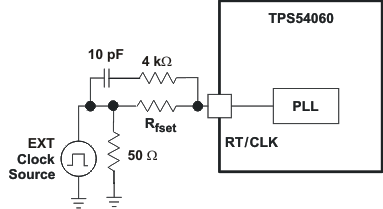Figure 46. Synchronizing to a System Clock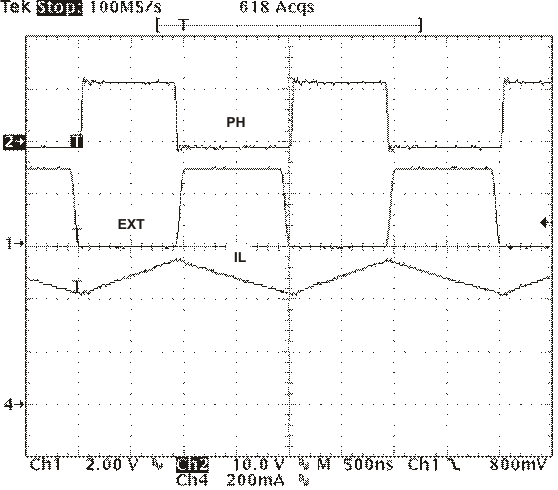Figure 47. Plot of Synchronizing in CCM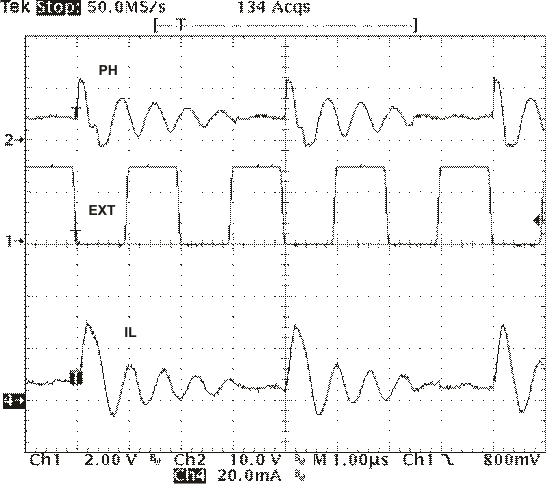Figure 49. Plot of Synchronizing in PSMFigure 48. Plot of Synchronizing in DCM

8.2 Typical Applications

8.2.1 Buck Converter for 3.3-V Output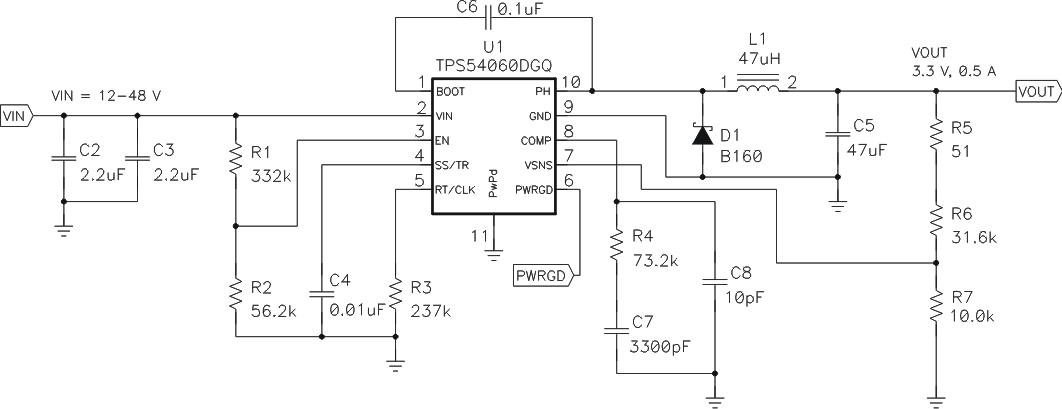Figure 50. High Frequency, 3.3V Output Power Supply Design with Adjusted UVLO

8.2.1.1 Design Requirements

This example details the design of a high frequency switching regulator design using ceramic output capacitors. A few parameters must be known in order to start the design process. These parameters are typically determined at the system level. For this example, we will start with the following known parameters:

Table 1. Design Parameters

DESIGN PARAMETERS EXAMPLE VALUES
Output Voltage 3.3 V
Transient Response 0 to 0.5-A load step ΔVout = 4%
Maximum Output Current 0.5 A
Input Voltage 34 V nom. 12 V to 48 V
Output Voltage Ripple 1% of Vout
Start Input Voltage (rising VIN) 8.9 V
Stop Input Voltage (falling VIN) 7.9 V

8.2.1.2.1 Selecting the Switching Frequency

The first step is to decide on a switching frequency for the regulator. Typically, the user will want to choose the highest switching frequency possible since this will produce the smallest solution size. The high switching frequency allows for lower valued inductors and smaller output capacitors compared to a power supply that switches at a lower frequency. The switching frequency that can be selected is limited by the minimum on-time of the internal power switch, the input voltage and the output voltage and the frequency shift limitation.

Equation 26 and Equation 27 must be used to find the maximum switching frequency for the regulator, choose the lower value of the two equations. Switching frequencies higher than these values will result in pulse skipping or the lack of overcurrent protection during a short circuit.

The typical minimum on time, tonmin, is 130 ns for the TPS54060. For this example, the output voltage is 3.3 V and the maximum input voltage is 48 V, which allows for a maximum switch frequency up to 616 kHz when including the inductor resistance, on resistance and diode voltage in Equation 26. To ensure overcurrent runaway is not a concern during short circuits in your design use Equation 27 or the solid curve in Figure 45 to determine the maximum switching frequency. With a maximum input voltage of 48 V, assuming a diode voltage of 0.5 V, inductor resistance of 130mΩ, switch resistance of 400mΩ, a current limit value of 0.94 A and a short circuit output voltage of 0.1V. The maximum switching frequency is approximately 923 kHz.

Choosing the lower of the two values and adding some margin a switching frequency of 500kHz is used. To determine the timing resistance for a given switching frequency, use Equation 2 or the curve in Figure 29.

The switching frequency is set by resistor R3 shown in Figure 50.

8.2.1.2.2 Output Inductor Selection (LO)

To calculate the minimum value of the output inductor, use Equation 28.

KIND is a coefficient that represents the amount of inductor ripple current relative to the maximum output current.

The inductor ripple current will be filtered by the output capacitor. Therefore, choosing high inductor ripple currents will impact the selection of the output capacitor since the output capacitor must have a ripple current rating equal to or greater than the inductor ripple current. In general, the inductor ripple value is at the discretion of the designer; however, the following guidelines may be used.

For designs using low ESR output capacitors such as ceramics, a value as high as KIND = 0.3 may be used. When using higher ESR output capacitors, KIND = 0.2 yields better results. Since the inductor ripple current is part of the PWM control system, the inductor ripple current should always be greater than 30 mA for dependable operation. In a wide input voltage regulator, it is best to choose an inductor ripple current on the larger side. This allows the inductor to still have a measurable ripple current with the input voltage at its minimum.

For this design example, use KIND = 0.3 and the minimum inductor value is calculated to be 39.7 μH. For this design, a nearest standard value was chosen: 47μH. For the output filter inductor, it is important that the RMS current and saturation current ratings not be exceeded. The RMS and peak inductor current can be found from Equation 30 and Equation 31.

For this design, the RMS inductor current is 0.501 A and the peak inductor current is 0.563 A. The chosen inductor is a MSS1048-473ML. It has a saturation current rating of 1.44 A and an RMS current rating of 1.83A.

As the equation set demonstrates, lower ripple currents will reduce the output voltage ripple of the regulator but will require a larger value of inductance. Selecting higher ripple currents will increase the output voltage ripple of the regulator but allow for a lower inductance value.

The current flowing through the inductor is the inductor ripple current plus the output current. During power up, faults or transient load conditions, the inductor current can increase above the calculated peak inductor current level calculated above. In transient conditions, the inductor current can increase up to the switch current limit of the device. For this reason, the most conservative approach is to specify an inductor with a saturation current rating equal to or greater than the switch current limit rather than the peak inductor current.

Equation 28.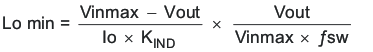Equation 29.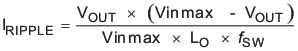Equation 30.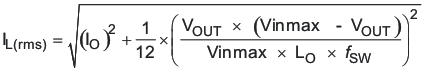Equation 31.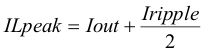8.2.1.2.3 Output Capacitor

There are three primary considerations for selecting the value of the output capacitor. The output capacitor will determine the modulator pole, the output voltage ripple, and how the regulators responds to a large change in load current. The output capacitance needs to be selected based on the more stringent of these three criteria.

The desired response to a large change in the load current is the first criteria. The output capacitor needs to supply the load with current when the regulator can not. This situation would occur if there are desired hold-up times for the regulator where the output capacitor must hold the output voltage above a certain level for a specified amount of time after the input power is removed. The regulator also will temporarily not be able to supply sufficient output current if there is a large, fast increase in the current needs of the load such as transitioning from no load to a full load. The regulator usually needs two or more clock cycles for the control loop to see the change in load current and output voltage and adjust the duty cycle to react to the change. The output capacitor must be sized to supply the extra current to the load until the control loop responds to the load change. The output capacitance must be large enough to supply the difference in current for 2 clock cycles while only allowing a tolerable amount of droop in the output voltage. Equation 32 shows the minimum output capacitance necessary to accomplish this.

For this example, the transient load response is specified as a 4% change in Vout for a load step from 0A (no load) to 0.5 A (full load). For this example, ΔIout = 0.5-0 = 0.5 A and ΔVout = 0.04 × 3.3 = 0.132 V. Using these numbers gives a minimum capacitance of 15.2μF. This value does not take the ESR of the output capacitor into account in the output voltage change. For ceramic capacitors, the ESR is usually small enough to ignore in this calculation. Aluminum electrolytic and tantalum capacitors have higher ESR that should be taken into account.

The catch diode of the regulator can not sink current so any stored energy in the inductor will produce an output voltage overshoot when the load current rapidly decreases, see Figure 51. The output capacitor must also be sized to absorb energy stored in the inductor when transitioning from a high load current to a lower load current. The excess energy that gets stored in the output capacitor will increase the voltage on the capacitor. The capacitor must be sized to maintain the desired output voltage during these transient periods. Equation 33 is used to calculate the minimum capacitance to keep the output voltage overshoot to a desired value. For this example, the worst case load step will be from 0.5 A to 0 A. The output voltage will increase during this load transition and the stated maximum in our specification is 4% of the output voltage. This will make Vf = 1.04 × 3.3 = 3.432. Vi is the initial capacitor voltage which is the nominal output voltage of 3.3 V. Using these numbers in Equation 33 yields a minimum capacitance of 13.2 μF.

Equation 34 calculates the minimum output capacitance needed to meet the output voltage ripple specification. Where fsw is the switching frequency, Voripple is the maximum allowable output voltage ripple, and Iripple is the inductor ripple current. Equation 34 yields 1μF.

Equation 35 calculates the maximum ESR an output capacitor can have to meet the output voltage ripple specification. Equation 35 indicates the ESR should be less than 248mΩ.

The most stringent criteria for the output capacitor is 15.2μF of capacitance to keep the output voltage in regulation during an load transient.

Additional capacitance de-ratings for aging, temperature and dc bias should be factored in which will increase this minimum value. For this example, a 47 μF 10V X5R ceramic capacitor with 5 mΩ of ESR will be used.

Capacitors generally have limits to the amount of ripple current they can handle without failing or producing excess heat. An output capacitor that can support the inductor ripple current must be specified. Some capacitor data sheets specify the Root Mean Square (RMS) value of the maximum ripple current. Equation 36 can be used to calculate the RMS ripple current the output capacitor needs to support. For this application, Equation 36 yields 37.7 mA.

Equation 32.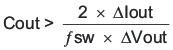where

• ΔIout is the change in output current.
• ƒsw is the regulators switching frequency.
• ΔVout is the allowable change in the output voltage.
Equation 33.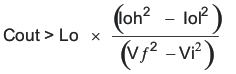where

• L is the value of the inductor.
• IOH is the output current under heavy load.
• IOL is the output under light load.
• VF is the final peak output voltage.
• Vi is the initial capacitor voltage.
Equation 34.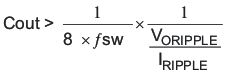Equation 35.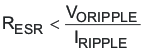Equation 36.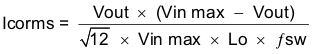8.2.1.2.4 Catch Diode

The TPS54060 requires an external catch diode between the PH pin and GND. The selected diode must have a reverse voltage rating equal to or greater than Vinmax. The peak current rating of the diode must be greater than the maximum inductor current. The diode should also have a low forward voltage. Schottky diodes are typically a good choice for the catch diode due to their low forward voltage. The lower the forward voltage of the diode, the higher the efficiency of the regulator.

Typically, the higher the voltage and current ratings the diode has, the higher the forward voltage will be. Since the design example has an input voltage up to 48V, a diode with a minimum of 60V reverse voltage will be selected.

For the example design, the B160A Schottky diode is selected for its lower forward voltage and it comes in a larger package size which has good thermal characteristics over small devices. The typical forward voltage of the B160A is 0.50 volts.

The diode must also be selected with an appropriate power rating. The diode conducts the output current during the off-time of the internal power switch. The off-time of the internal switch is a function of the maximum input voltage, the output voltage, and the switching frequency. The output current during the off-time is multiplied by the forward voltage of the diode which equals the conduction losses of the diode. At higher switch frequencies, the ac losses of the diode need to be taken into account. The ac losses of the diode are due to the charging and discharging of the junction capacitance and reverse recovery. Equation 37 is used to calculate the total power dissipation, conduction losses plus ac losses, of the diode.

The B160A has a junction capacitance of 110pF. Using Equation 37, the selected diode will dissipate 0.297 Watts. This power dissipation, depending on mounting techniques, should produce a 5.9°C temperature rise in the diode when the input voltage is 48V and the load current is 0.5A.

If the power supply spends a significant amount of time at light load currents or in sleep mode consider using a diode which has a low leakage current and slightly higher forward voltage drop.

Equation 37.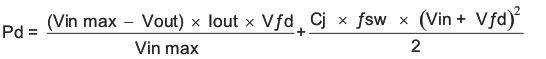8.2.1.2.5 Input Capacitor

The TPS54060 requires a high quality ceramic, type X5R or X7R, input decoupling capacitor of at least 3 μF of effective capacitance and in some applications a bulk capacitance. The effective capacitance includes any dc bias effects. The voltage rating of the input capacitor must be greater than the maximum input voltage. The capacitor must also have a ripple current rating greater than the maximum input current ripple of the TPS54060. The input ripple current can be calculated using Equation 38.

The value of a ceramic capacitor varies significantly over temperature and the amount of dc bias applied to the capacitor. The capacitance variations due to temperature can be minimized by selecting a dielectric material that is stable over temperature. X5R and X7R ceramic dielectrics are usually selected for power regulator capacitors because they have a high capacitance to volume ratio and are fairly stable over temperature. The output capacitor must also be selected with the dc bias taken into account. The capacitance value of a capacitor decreases as the dc bias across a capacitor increases.

For this example design, a ceramic capacitor with at least a 60V voltage rating is required to support the maximum input voltage. Common standard ceramic capacitor voltage ratings include 4V, 6.3V, 10V, 16V, 25V, 50V or 100V so a 100V capacitor should be selected. For this example, two 2.2μF, 100V capacitors in parallel have been selected. Table 2 shows a selection of high voltage capacitors. The input capacitance value determines the input ripple voltage of the regulator. The input voltage ripple can be calculated using Equation 39. Using the design example values, Ioutmax = 0.5 A, Cin = 4.4μF, ƒsw = 500 kHz, yields an input voltage ripple of 57 mV and a rms input ripple current of 0.223A.

Equation 38.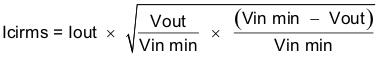Equation 39.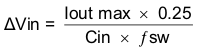Table 2. Capacitor Types

VENDOR VALUE (μF) EIA Size VOLTAGE DIALECTRIC COMMENTS
Murata 1.0 to 2.2 1210 100 V X7R GRM32 series
1.0 to 4.7 50 V
1.0 1206 100 V GRM31 series
1.0 to 2.2 50 V
Vishay 1.0 10 1.8 2220 50 V VJ X7R series
1.0 to 1.2 100 V
1.0 to 3.9 2225 50 V
1.0 to 1.8 100 V
TDK 1.0 to 2.2 1812 100 V C series C4532
1.5 to 6.8 50 V
1.0. to 2.2 1210 100 V C series C3225
1.0 to 3.3 50 V
AVX 1.0 to 4.7 1210 50 V X7R dielectric series
1.0 100 V
1.0 to 4.7 1812 50 V
1.0 to 2.2 100 V

8.2.1.2.6 Slow Start Capacitor

The slow start capacitor determines the minimum amount of time it will take for the output voltage to reach its nominal programmed value during power up. This is useful if a load requires a controlled voltage slew rate. This is also used if the output capacitance is large and would require large amounts of current to quickly charge the capacitor to the output voltage level. The large currents necessary to charge the capacitor may make the TPS54060 reach the current limit or excessive current draw from the input power supply may cause the input voltage rail to sag. Limiting the output voltage slew rate solves both of these problems.

The slow start time must be long enough to allow the regulator to charge the output capacitor up to the output voltage without drawing excessive current. Equation 40 can be used to find the minimum slow start time, tss, necessary to charge the output capacitor, Cout, from 10% to 90% of the output voltage, Vout, with an average slow start current of Issavg. In the example, to charge the 47μF output capacitor up to 3.3V while only allowing the average input current to be 0.125A would require a 1 ms slow start time.

Once the slow start time is known, the slow start capacitor value can be calculated using Equation 1. For the example circuit, the slow start time is not too critical since the output capacitor value is 47μF which does not require much current to charge to 3.3V. The example circuit has the slow start time set to an arbitrary value of 3.2ms which requires a 0.01 μF capacitor.

Equation 40.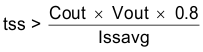8.2.1.2.7 Bootstrap Capacitor Selection

A 0.1-μF ceramic capacitor must be connected between the BOOT and PH pins for proper operation. It is recommended to use a ceramic capacitor with X5R or better grade dielectric. The capacitor should have a 10V or higher voltage rating.

8.2.1.2.8 Undervoltage Lockout (UVLO) Set Point

The UVLO can be adjusted using an external voltage divider on the EN pin of the TPS54060. The UVLO has two thresholds, one for power up when the input voltage is rising and one for power down or brown outs when the input voltage is falling. For the example design, the supply should turn on and start switching once the input voltage increases above 8.9V (enabled). After the regulator starts switching, it should continue to do so until the input voltage falls below 7.9V (UVLO stop).

The programmable UVLO and enable voltages are set using a resistor divider between Vin and ground to the EN pin. Equation 18 through Equation 19 can be used to calculate the resistance values necessary. For the example application, a 332kΩ between Vin and EN and a 56.2kΩ between EN and ground are required to produce the 8.9 and 7.9 volt start and stop voltages.

8.2.1.2.9 Output Voltage and Feedback Resistors Selection

For the example design, 10.0 kΩ was selected for R2. Using Equation 17, R1 is calculated as 31.25 kΩ. The nearest standard 1% resistor is 31.6 kΩ. Due to current leakage of the VSENSE pin, the current flowing through the feedback network should be greater than 1 μA in order to maintain the output voltage accuracy. This requirement makes the maximum value of R2 equal to 800 kΩ. Choosing higher resistor values will decrease quiescent current and improve efficiency at low output currents but may introduce noise immunity problems.

8.2.1.2.10 Compensation

There are several methods used to compensate DC/DC regulators. The method presented here is easy to calculate and ignores the effects of the slope compensation that is internal to the device. Since the slope compensation is ignored, the actual cross over frequency will usually be lower than the cross over frequency used in the calculations. This method assume the crossover frequency is between the modulator pole and the esr zero and the esr zero is at least 10 times greater the modulator pole. Use SwitcherPro software for a more accurate design.

To get started, the modulator pole, fpmod, and the esr zero, fz1 must be calculated using Equation 41 and Equation 42. For Cout, use a derated value of 40 μF. Use equations Equation 43 and Equation 44, to estimate a starting point for the crossover frequency, fco, to design the compensation. For the example design, fpmod is 603 Hz and fzmod is 796 kHz. Equation 43 is the geometric mean of the modulator pole and the esr zero and Equation 44 is the mean of modulator pole and the switching frequency. Equation 43 yields 21.9 kHz and Equation 44 gives 12.3 kHz. Use the lower value of Equation 43 or Equation 44 for an initial crossover frequency. For this example, fco is 12.3kHz. Next, the compensation components are calculated. A resistor in series with a capacitor is used to create a compensating zero. A capacitor in parallel to these two components forms the compensating pole.

Equation 41.Equation 42.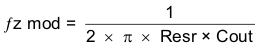Equation 43.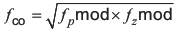Equation 44.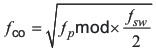To determine the compensation resistor, R4, use Equation 45. Assume the power stage transconductance, gmps, is 1.9A/V. The output voltage, Vo, reference voltage, VREF, and amplifier transconductance, gmea, are 3.3V, 0.8V and 97μS, respectively. R4 is calculated to be 72.6 kΩ, use the nearest standard value of 73.2kΩ. Use Equation 46 to set the compensation zero to the modulator pole frequency. Equation 46 yields 3600pF for compensating capacitor C7, a 3300pF is used on the board.

Equation 45.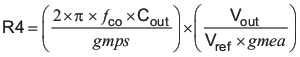Equation 46.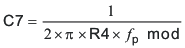Use the larger value of Equation 47 and Equation 48 to calculate the C8, to set the compensation pole. Equation 48 yields 8.7pF so the nearest standard of 10pF is used.

Equation 47.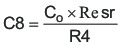Equation 48.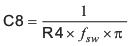8.2.1.2.11 Discontinuous Mode and Eco Mode Boundary

With an input voltage of 34V, the power supply enters discontinuous mode when the output current is less than 60mA. The power supply enters EcoMode when the output current is lower than 38mA.

The input current draw at no load is 228μA.

8.2.1.2.12 Power Dissipation Estimate

The following formulas show how to estimate the IC power dissipation under continuous conduction mode (CCM) operation. These equations should not be used if the device is working in discontinuous conduction mode (DCM).

The power dissipation of the IC includes conduction loss (Pcon), switching loss (Psw), gate drive loss (Pgd) and supply current (Pq).

Equation 49.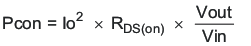Equation 50.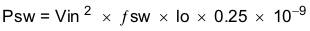Equation 51.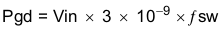Equation 52.Where:

Io is the output current (A).
RDS(on) is the on-resistance of the high-side MOSFET (Ω).
VOUT is the output voltage (V).
VIN is the input voltage (V).
fsw is the switching frequency (Hz).

So

Equation 53.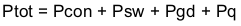For given TA,

Equation 54.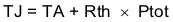For given TJMAX = 150°C

Equation 55.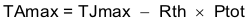Where:

Ptot is the total device power dissipation (W).
TA is the ambient temperature (°C).
TJ is the junction temperature (°C).
Rth is the thermal resistance junction to ambient for a given PCB layout (°C/W).
TJMAX is maximum junction temperature (°C).
TAMAX is maximum ambient temperature (°C).

There will be additional power losses in the regulator circuit due to the inductor ac and dc losses, the catch diode and trace resistance that will impact the overall efficiency of the regulator.

8.2.1.3 Application Curves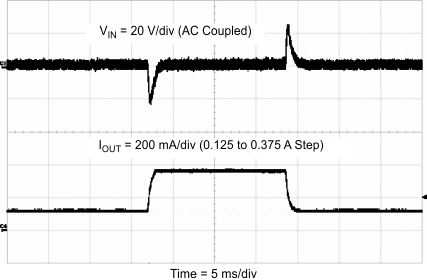Figure 51. Load Transient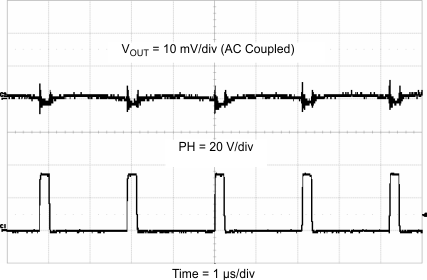Figure 53. Output Ripple CCM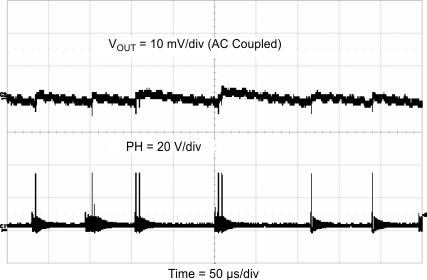Figure 55. Output Ripple, PSM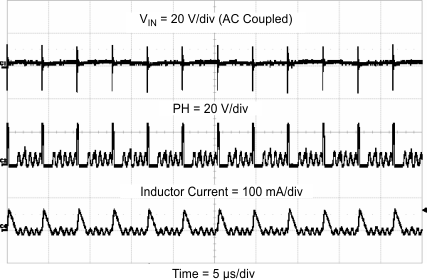Figure 57. Input Ripple DCM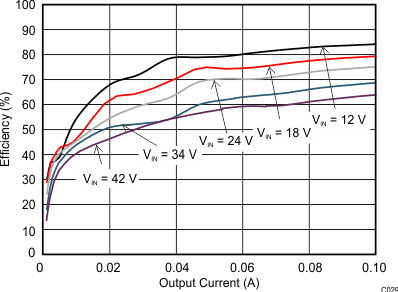Figure 59. Light Load Efficiency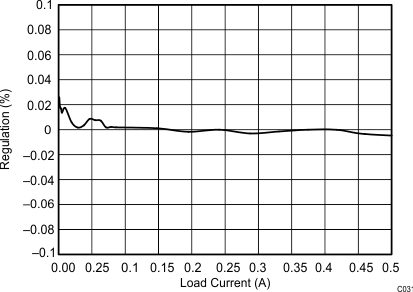Figure 61. Regulation vs Load Current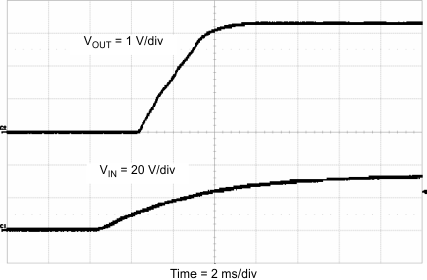Figure 52. Start-Up With VIN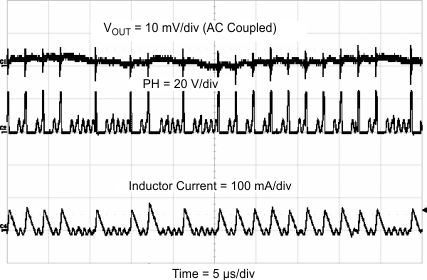Figure 54. Output Ripple, DCM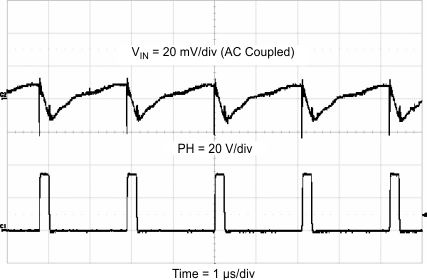Figure 56. Input Ripple CCM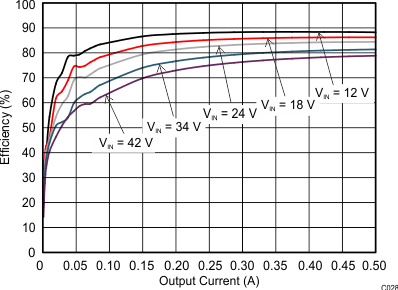Figure 58. Efficiency vs Load Current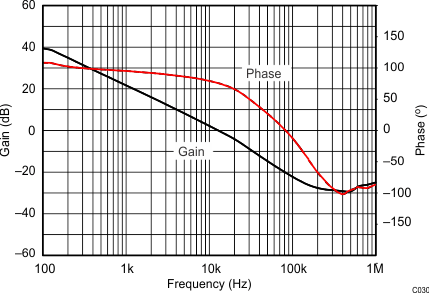Figure 60. Overall Loop Frequency Response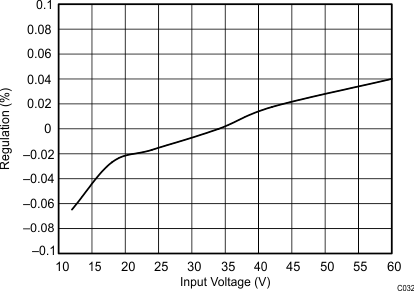Figure 62. Regulation vs Input Voltage

8.2.2 Inverting Power Supply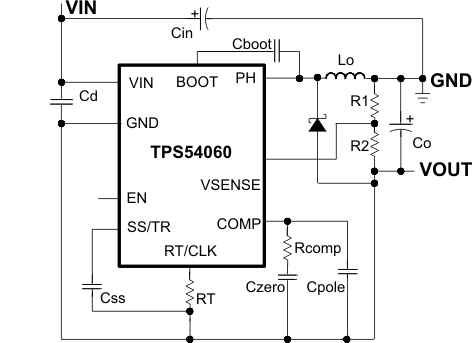Figure 63. Inverting Power Supply from the SLVA317 Application Note

8.2.3 Split Rail Power Supply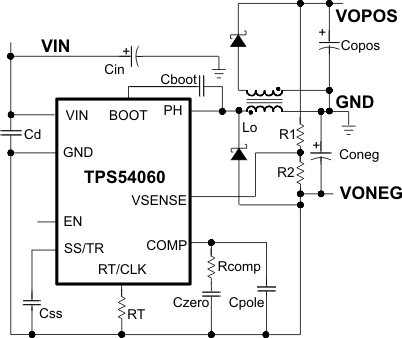Figure 64. Split Rail Power Supply Based on the SLVA369 Application Note